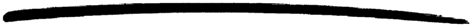## 5 Functions

### 5.1 Function Declaration Syntax

A function declaration is a sequence of function clauses separated by semicolons, and terminated by period (.).

A function clause consists of a clause head and a clause body, separated by `->`.

A clause head consists of the function name, an argument list, and an optional guard sequence beginning with the keyword `when`.

```Name(Pattern11,...,Pattern1N) [when GuardSeq1] ->
Body1;
...;
Name(PatternK1,...,PatternKN) [when GuardSeqK] ->
BodyK.

```

The function name is an atom. Each argument is a pattern.

The number of arguments `N` is the arity of the function. A function is uniquely defined by the module name, function name and arity. That is, two functions with the same name and in the same module, but with different arities are two completely different functions.

A function named `f` in the module `m` and with arity `N` is often denoted as `m:f/N`.

A clause body consists of a sequence of expressions separated by comma (,):

```Expr1,
...,
ExprN

```

Valid Erlang expressions and guard sequences are described in Erlang Expressions.

Example:

```fact(N) when N>0 ->  % first clause head
N * fact(N-1);   % first clause body

fact(0) ->           % second clause head
1.               % second clause body

```

### 5.2 Function Evaluation

When a function `m:f/N` is called, first the code for the function is located. If the function cannot be found, an `undef` run-time error will occur. Note that the function must be exported to be visible outside the module it is defined in.

If the function is found, the function clauses are scanned sequentially until a clause is found that fulfills the following two conditions:

1. the patterns in the clause head can be successfully matched against the given arguments, and
2. the guard sequence, if any, is true.

If such a clause cannot be found, a `function_clause` run-time error will occur.

If such a clause is found, the corresponding clause body is evaluated. That is, the expressions in the body are evaluated sequentially and the value of the last expression is returned.

Example: Consider the function `fact`:

```-module(m).
-export([fact/1]).

fact(N) when N>0 ->
N * fact(N-1);
fact(0) ->
1.

```

Assume we want to calculate factorial for 1:

```1> m:fact(1).

```

Evaluation starts at the first clause. The pattern `N` is matched against the argument 1. The matching succeeds and the guard (`N>0`) is true, thus `N` is bound to 1 and the corresponding body is evaluated:

```N * fact(N-1) => (N is bound to 1)
1 * fact(0)

```

Now `fact(0)` is called and the function clauses are scanned sequentially again. First, the pattern `N` is matched against 0. The matching succeeds, but the guard (`N>0`) is false. Second, the pattern 0 is matched against 0. The matching succeeds and the body is evaluated:

```1 * fact(0) =>
1 * 1 =>
1

```

Evaluation has succeed and `m:fact(1)` returns 1.

If `m:fact/1` is called with a negative number as argument, no clause head will match. A `function_clause` run-time error will occur.

### 5.3 Tail recursion

If the last expression of a function body is a function call, a tail recursive call is done so that no system resources for example call stack are consumed. This means that an infinite loop can be done if it uses tail recursive calls.

Example:

```loop(N) ->
io:format("~w~n", [N]),
loop(N+1).

```

As a counter-example see the factorial example above that is not tail recursive since a multiplication is done on the result of the recursive call to `fact(N-1)`.

### 5.4 Built-In Functions, BIFs

Built-in functions, BIFs, are implemented in C code in the runtime system and do things that are difficult or impossible to implement in Erlang. Most of the built-in functions belong to the module `erlang` but there are also built-in functions belonging to a few other modules, for example `lists` and `ets`.

The most commonly used BIFs belonging to `erlang` are auto-imported, they do not need to be prefixed with the module name. Which BIFs are auto-imported is specified in `erlang(3)`. For example, standard type conversion BIFs like `atom_to_list` and BIFs allowed in guards can be called without specifying the module name. Examples:

```1> size({a,b,c}).
3
2> atom_to_list('Erlang').
"Erlang"

```

Note that normally it is the set of auto-imported built-in functions that is referred to when talking about 'BIFs'.